Test: Two Port Network - 1

# Test: Two Port Network - 1

Test Description

## 10 Questions MCQ Test Network Theory (Electric Circuits) | Test: Two Port Network - 1

Test: Two Port Network - 1 for Electrical Engineering (EE) 2023 is part of Network Theory (Electric Circuits) preparation. The Test: Two Port Network - 1 questions and answers have been prepared according to the Electrical Engineering (EE) exam syllabus.The Test: Two Port Network - 1 MCQs are made for Electrical Engineering (EE) 2023 Exam. Find important definitions, questions, notes, meanings, examples, exercises, MCQs and online tests for Test: Two Port Network - 1 below.
Solutions of Test: Two Port Network - 1 questions in English are available as part of our Network Theory (Electric Circuits) for Electrical Engineering (EE) & Test: Two Port Network - 1 solutions in Hindi for Network Theory (Electric Circuits) course. Download more important topics, notes, lectures and mock test series for Electrical Engineering (EE) Exam by signing up for free. Attempt Test: Two Port Network - 1 | 10 questions in 30 minutes | Mock test for Electrical Engineering (EE) preparation | Free important questions MCQ to study Network Theory (Electric Circuits) for Electrical Engineering (EE) Exam | Download free PDF with solutions
 1 Crore+ students have signed up on EduRev. Have you?
Test: Two Port Network - 1 - Question 1

### [T] = ?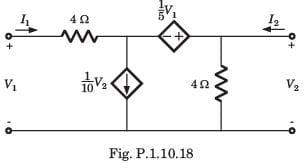Detailed Solution for Test: Two Port Network - 1 - Question 1

Let I3 be the clockwise loop current in center loop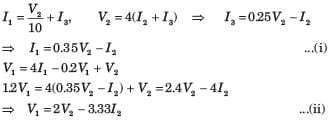Test: Two Port Network - 1 - Question 2

### [h] = ?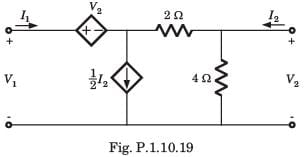Detailed Solution for Test: Two Port Network - 1 - Question 2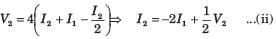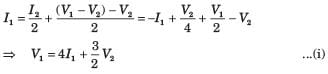Test: Two Port Network - 1 - Question 3

### [y] = ?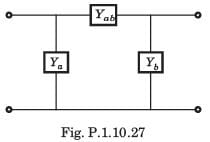Detailed Solution for Test: Two Port Network - 1 - Question 3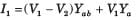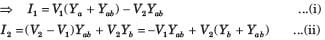Test: Two Port Network - 1 - Question 4

The y-parameters of a 2-port network are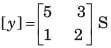A resistor of 1 ohm is connected across as shown in fig. P.1.10.2 8. The new y –parameter would be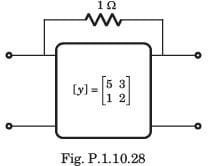Detailed Solution for Test: Two Port Network - 1 - Question 4

y-parameter of 1Ω resistor network are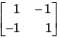New y-parameter =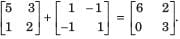Test: Two Port Network - 1 - Question 5

For the 2-port of fig. P.1.10.29,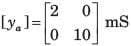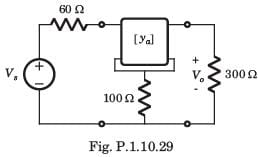The value of Vo/Vs is

Detailed Solution for Test: Two Port Network - 1 - Question 5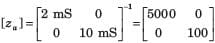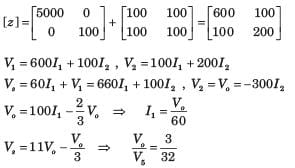Test: Two Port Network - 1 - Question 6

The T-parameters of a 2-port network are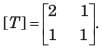If such two 2-port network are cascaded, the z –parameter for the cascaded network is

Detailed Solution for Test: Two Port Network - 1 - Question 6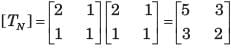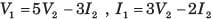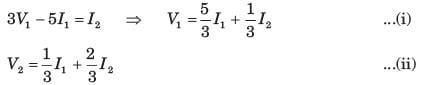Test: Two Port Network - 1 - Question 7

[y] = ?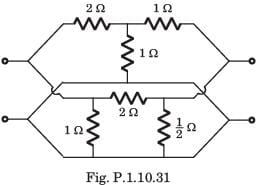Detailed Solution for Test: Two Port Network - 1 - Question 7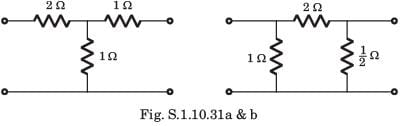Test: Two Port Network - 1 - Question 8

[y] = ?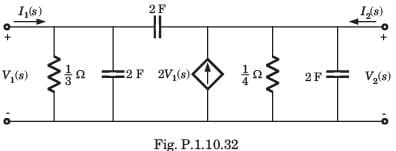Detailed Solution for Test: Two Port Network - 1 - Question 8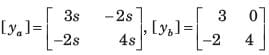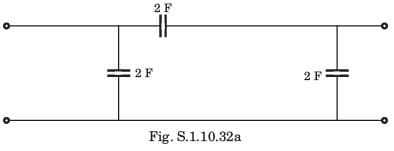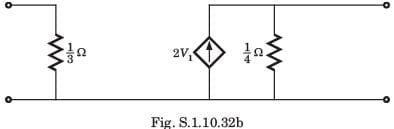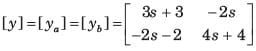Test: Two Port Network - 1 - Question 9

h21 = ?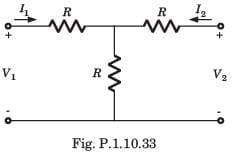Detailed Solution for Test: Two Port Network - 1 - Question 9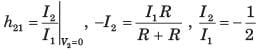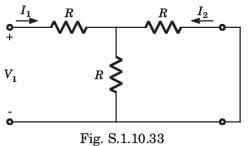Test: Two Port Network - 1 - Question 10

In the circuit shown in fig. P.1.10.34, when the voltage V1 is 10 V, the current I is 1 A. If the applied voltage at port-2 is 100 V, the short circuit current flowing through at port 1 will be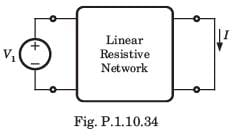Detailed Solution for Test: Two Port Network - 1 - Question 10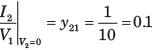Interchanging the port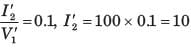## Network Theory (Electric Circuits)

23 videos|63 docs|61 tests
Information about Test: Two Port Network - 1 Page
In this test you can find the Exam questions for Test: Two Port Network - 1 solved & explained in the simplest way possible. Besides giving Questions and answers for Test: Two Port Network - 1 , EduRev gives you an ample number of Online tests for practice

## Network Theory (Electric Circuits)

23 videos|63 docs|61 tests(Scan QR code)